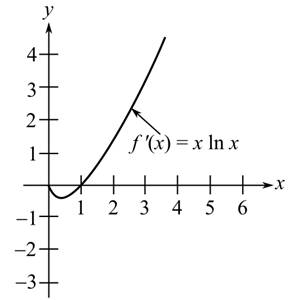# The slope for the function f at the point x = 2 where f ' is represented by the graph as follows:### Calculus

10th Edition
Ron Larson + 1 other
Publisher: Cengage Learning
ISBN: 9781285057095### Calculus

10th Edition
Ron Larson + 1 other
Publisher: Cengage Learning
ISBN: 9781285057095

#### Solutions

Chapter 8.2, Problem 62E

(a)

To determine

## To calculate: The slope for the function f at the point x=2 where f' is represented by the graph as follows:(b)

To determine

### Want to see the full answer?

Check out a sample textbook solution.

### Want to see this answer and more?

Experts are waiting 24/7 to provide step-by-step solutions in as fast as 30 minutes!*

*Response times may vary by subject and question complexity. Median response time is 34 minutes for paid subscribers and may be longer for promotional offers.